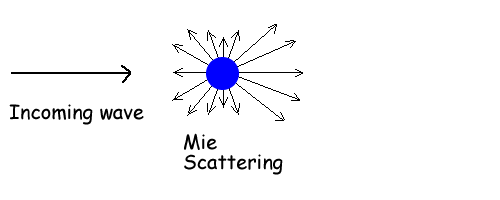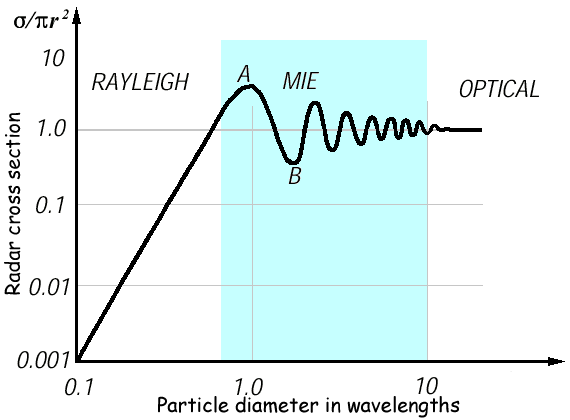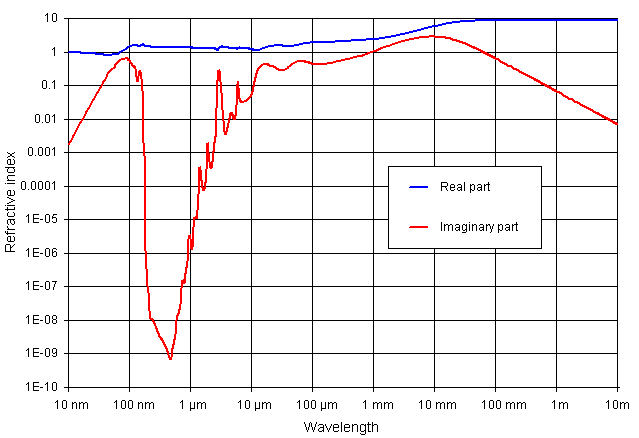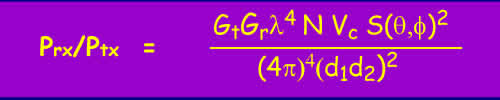This section deals with the extended propagation of microwaves via scatter from rain and clouds

The Detailed propagation course

Short and simple (the old tutorial)

We have already discussed the scattering of radiowaves by irregularities within the troposphere. Another effect that is particularly important above 3GHz is Rainscatter - or more strictly Hydrometeor scatter. Hydrometeors are essentially particles of water within the atmosphere, they can take the form of liquid water as in rain, mist and fog or ice as in clouds, hail and snow.

Rainscatter can extend the propagation of microwave signals to well beyond line of sight. With scattering from rain at 2-4km above the ground, the range extension can be by several hundred km. A site that estimates rain scatter for amatuer radio purposes based on weather radar is currently available at http://www.frontiernet.net/~aflowers/rainscatter/ .

The forward scatter by Hydrometeors at microwaves is via a mechanism called Rayleigh scattering. Rayleigh wrote a paper about it in 1871, hence the name. Rayleigh type scattering occurs when the particle is electrically small and phase shift across the particle is small, that is the particle is much smaller than the wavelength. Rayleigh scattering scatters energy with a radiation pattern like that of a dipole. If the particle is of the order of the size of the wavelength, Mie scattering occurs. With Mie scattering, there is more forward scattering and a forward radiation lobe.The Rayleigh and Mie are not different mechanisms, they are simply models approximating the scattering mechanism applicable at different wavelengths. The following diagram from a radar discussion demonstrates this well.Scattering does not just affect radiowaves, scattering of light by molecules in the air is why the sky is not black. There is a wavelength dependence inversely proportional to the 4th power, shorter wavelengths are scattered more.

A practical example is the very small particles in the upper atmosphere scatter blue light more than red light because blue light has a shorter wavelength, hence the sky is blue. The very small water particles in clouds are still much larger than the wavelength of light, so there is no frequency selectivity and the scatterd light is white. Large raindrops allow light to be internally reflected, with the refracted angle dependent on wavelength, hence the rainbow.

This wavelength dependence is also why VHF and UHF signals are not significantly scattered whereas SHF and up signals are. Most of the scattering from rain for microwave signals can be considered to be Rayleigh scattering. The Rayleigh criterion:

pD/l <<1 where D is the diameter of the particle and

pnD/l <<1 where n is the refractive index.

If the above are true, the Rayleigh approximation can be made. A typical raindrop may have a diameter of 1mm so assuming a refractive index of 1 (see later) pnD/l = 1 if l ~ 3mm equivalent to around 100GHz. So for this 1mm raindrop if the frequency is below 100GHz we will observe Rayleigh scattering and scattered electrical field will be like that of a dipole.

Now we get to the maths...(Skip the maths). The general scattering function is calculable via electromagnetic theory beyond the scope of this tutorial but it is important to know what it relates to, which is the ratio of the scattered to the incident field strength at some point defined in 3D space - usually using spherical co-ordinates (r, q, f). The most interesting is the forward scattering function So- which defines how much is energy scattered in the same direction the incoming wave was originally going. A parameter often mentioned is the extinction cross section of a particle - which is related to the real part (the power, not phase) of So.

Cext = l2/ p Re[So]

This is a useful parameter with units of area that can be used to calculate both scattered signals and attenuation as a result of energy lost through scattering. If our radiowave is propagating through a region containing scattering particles the attenuation resulting through scattering loss is related to the product of the particle density N (units of particles per unit volume) and the extinction cross section Cext (units of area).

If the Rayleigh criterion is satisfied, the dipole moment P is related to an incident electric field can be written as:

P = eo(er -1)zv = eoUv

Where v is the volume of the particle and z is the ratio of the internal and external field 3/(er + 2) for a sphere. The parameter U is tabulated below for common Hydrometeors depending on whether the field is parallel or perpendicular to the longest axis of the particle. The power re-radiated (scattered) by this dipole is:

P2/3eo2l4 = (Uv)2/3l4

Note the dependence with 1/l4 which explains why the scattered energy increases rapidly as the wavelength decreases. Note also that some power is absorbed , the imaginary part of So. Rain attenuation is a mixture of scatter loss and absorption.

 Water Sphere (raindrop) Ice Sphere (Hail) Ice Needle Ice Plate U parallel 3(er - 1)/(er + 2) ~ 3 1.26 2.32 2.32 U perpendicular 3(er - 1)/(er + 2) ~ 3 1.26 1.04 0.68

The Refractive Index of Water and Ice

The refractive index of water is rather complicated with a strong temperature and frequency dependence. The real part is around 10 up to 30GHz with the imaginary part varying from around 0.2 to 2 but generally rising with temperature and frequency. Above 30GHz both the real and imaginary parts fall. http://www.philiplaven.com/p20.html gives an excellent summary.The low value of the imaginary part of the refractive index of water in the region 1mm to 100nm indicates that water will be transparent at optical frequencies, as we all know, it is.

The real part of the refractive index of ice is around 1.78 up to 300GHz with a small imaginary component (loss tangent) that falls with frequency. This is incidentally why microwave ovens are not good at melting ice.

In order to calculate the scattered energy from a particular rain cell, it is necessary to use the bistatic radar equation which was derived in the section on reflections.The reflection area A in the above equation is replaced by an expression for the rain/ice scatter.where N is the particle density, Vc is the "common volume" of the scattering region formed by the rain/ice and the intersection of the antenna beams and S(q,f) is the scattering function. At the extremes of range, S(q,f) can be replaced by So which we can calculate from the Extinction Cross Section Cext of the particles. Cext = l2/p Re[So]. The extinction cross section increases approximately in proportion to the third power of the drop size and with the inverse square of the wavelength, i.e. D3/l2in the Rayleigh region.

Essential Features of Rain Scatter

Naturally with N and Vc being weather dependent, values vary considerably with time and space - so actually calculating the rainscatter signal requires complex integration, assuming the structure of the rain is known or can be modelled and will generally require a numerical integration using a computer programme. However, from the above equations we can identify the important features are:

that the received signal strength is proportional to:

• transmitted power (obviously)
• the common volume (this is the antenna beam intersection),
• the particle density (for example how hard it is raining)
• the scattering function So2 (how well the particles scatter - for example how large they are, D6)

and is inversely proportional to:

• the 4th power of range
• the 4th power of the wavelength.

Of course, the rain also attenuates the energy in the radiowave, so the scattered power at the receiver will be reduced.

Some typical values

N will be a distribution of drop sizes ranging from 0.1mm to 6mm with the most common sizes being 1-2mm with the density depending on the rainfall rate, the height of common volume and whether or not this includes the melting layer. A typical value for Cext are shown in the table.

 Freq/Size 0.5mm 1mm 2mm 3mm 6mm 3GHz --- 0.001 0.01 0.04 1.0 10GHz 0.0015 0.015 0.4 1.0 30 24GHz 0.01 0.2 3 15 60

Cext vs Frequency and Drop size

Where is there liquid water in the air?

Much of the water that is in the air is in the form of water vapour rather than as a liquid or solid hydrometeors. As water freezes at 0C, where the air temperature is below 0C the predominant hydrometeors are ice. At just below the height where the temperature is 0C this ice melts and falls as rain - though it is nothing like as simple as that in practice. Ice and liquid water are both heavier then air and the only thing stopping them falling to the ground are rising air currents. As water condenses out of the air it forms ice crystals which are small enough to be supported by rising air currents. These particles clump together and get larger until they are too heavy for air currents to support. These heavy particles fall as rain, hail or snow. There is a fair amount of turbulence associated with clouds and the region where ice is melting into rain and forming large particles is a particularly good scatterer of microwave energy. In weather radars it is described as the bright band as it produces a strong return echo.

© Mike Willis December 26th, 2006